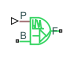# Rotational Velocity Noise Source

Produce zero-mean normally (Gaussian) distributed rotational velocity

•Libraries:
Simscape / Driveline / Sources

## Description

The Rotational Velocity Source block generates zero-mean normally (Gaussian) distributed rotational velocity using the PS Random Number source in the Simscape™ Foundation library. The rotational velocity produced by the block depends on two factors:

• The noise power spectral density input to the block

• A random number seed that you control using the Repeatability and, as applicable, Seed parameters

The defining equation for the rotational velocity that the block generates is

`$v=\sqrt{PSD/2}\frac{N\left(0,1\right)}{\sqrt{h}},$`

where:

• v is the output rotational velocity.

• PSD is the single-sided noise power spectral density.

• N is a Gaussian random number with zero mean and standard deviation of one.

• h is the sampling interval.

## Ports

### Input

expand all

The power spectral density (PSD) of noise is the average noise power per unit of bandwidth, in (rad/s)2/Hz.

### Conserving

expand all

Mechanical rotational conserving port associated with the base interface.

Mechanical rotational conserving port associated with the follower interface.

## Parameters

expand all

The values of the time step period and the initial time offset. If you specify a scalar value for step, the block assumes an offset value of `0`.To specify a nonzero value for the initial time offset, specify the parameter values using the vector ```[step, offset]```. The offset value must be less than the step value and greater than or equal to zero.

The random number seed is the number that initializes the random number generator. The seed is `0` or a positive integer. To control the random number seed, set this parameter to:

• `Not repeatable` — The seed changes every time you simulate your model. The block resets the random seed using the MATLAB® random number generator command:

`seed = randi(2^32-1);`
• `Repeatable` — The seed is the same random number at the start of every simulation. The block sets the value using the same MATLAB random number generator command used by the `Not repeatable` parameter.

When you add a Rotational Velocity Noise Source block to your model from the Sources library, the block generates and stores a random value for the repeated seed. When you make a copy of the Rotational Velocity Noise Source block from an existing block in a model, the copy generates a new random value for the repeated seed.

• `Specify seed` — The seed is a number that you specify using the Seed parameter. The Seed parameter is only available when you choose ```Specify seed``` for the Repeatability parameter.

The seed must be `0` or a positive integer. This parameter is only available when you select ```Specify seed``` for the Repeatability parameter.

## Version History

Introduced in R2016b